## Semaphore in OS-

Before you go through this article, make sure that you have gone through the previous article on Semaphores in OS.

We have discussed-

• A semaphore is a simple integer variable used to provide synchronization among the processes.
• There are mainly two types of semaphores-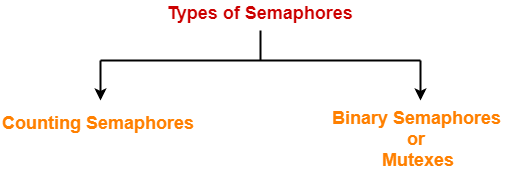In this article, we will discuss practice problems based on Binary Semaphores.

## Problem-01:

Each process Pi, i = 1, 2, …, 9 is coded as follows-

```repeat
P(mutex)
{ Critical Section }
V(mutex)
forever```

The code for P10 is identical except that it uses V(mutex) in place of P(mutex). What is the largest number of processes that can be inside the critical section at any moment?

1. 1
2. 2
3. 3
4. None of these

## Solution-

### Code for Processes P1, P2, … , P9

```repeat
P(mutex)
{ Critical Section }
V(mutex)
forever```

### Code for Process P10

```repeat
V(mutex)
{ Critical Section }
V(mutex)
forever```

Consider mutex is initialized with 1.

Then-

• All the 10 processes may be present inside the critical section at the same time.
• If there would have been more number of processes, then they could also be present inside the critical section at the same time.

The explanation is as follows-

## Explanation-

### Scene-01:

• Initially, process P1 arrives.
• It performs the wait operation on mutex and sets its value to 0.
• It then enters the critical section.

### Scene-02:

• Consider the processes P2, P3, … , P9 arrives when process P1 is executing the critical section.
• All the other processes get blocked and are put to sleep in the waiting list.

### Scene-03:

• Process P10 arrives.
• It performs the signal operation on mutex.
• It selects one process from the waiting list say process P2 and wakes it up.
• Process P2 can now enter the critical section (P1 is already present).
• Now, process P10 enters the critical section (P1 and P2 are already present).
• After executing critical section, during exit, it again performs the signal operation on mutex.
• It selects one process from the waiting list say process P3 and wakes it up.
• Process P3 can now enter the critical section (P1 and P2 are already present).
• Process P10 may keep on executing repeatedly.
• It wakes up 2 processes each time during its course of execution.
• In this manner, all the processes blocked in the waiting list may get entry inside the critical section.

Thus, Option (D) is correct.

## Problem-02:

Let m…m be mutexes (binary semaphores) and P…P be processes. Suppose each process P[i] executes the following:

wait(m[i]);

wait(m[(i+1) mod 4]);

………….

release(m[i]);

release(m[(i+1) mod 4]);

This could cause-

1. Thrashing
4. None of the above

## Solution-

Given processes have to execute the wait operations as-

• Process P0 : wait(m); wait(m);
• Process P1 : wait(m); wait(m);
• Process P2 : wait(m); wait(m);
• Process P3 : wait(m); wait(m);
• Process P4 : wait(m); wait(m);

Now,

• A deadlock may be caused when different processes execute the wait operations on mutexes.
• The occurrence of following scenes will give birth to a deadlock.

### Scene-01:

• Process P0 arrives.
• It executes wait(m) and gets preempted.

### Scene-02:

• Process P1 gets scheduled.
• It executes wait(m) and gets preempted.

### Scene-03:

• Process P2 gets scheduled.
• It executes wait(m) and gets preempted.

### Scene-04:

• Process P3 gets scheduled.
• It executes wait(m) and gets preempted.

### Scene-05:

• Process P4 gets scheduled.
• It executes wait(m) and gets preempted.

Now,

• The system is in a deadlock state since no process can proceed its execution.
• Thus, Option (B) is correct.

## Problem-03:

In the above question, which of the following pairs of processes may be present inside the critical section at the same time?

1. (P0, P2)
2. (P1, P3)
3. (P2, P4)
4. (P3, P4)
5. All of these

## Solution-

• All the given pair of processes in options execute wait operation on different mutexes.
• Hence, they can get entry inside the critical section at the same time.
• Thus, Option (E) is correct.

### NOTE-

• Mutual exclusion is violated here.
• Maximum number of processes that may be present inside the critical section at the same time = 2.

## Problem-04:

The following program consists of 3 concurrent processes and 3 binary semaphores. The semaphores are initialized as S0 = 1, S1 = 0 and S2 = 0.

 Process P0 Process P1 Process P2 while (true) { wait (S0); print ‘0’ release (S1); release (S2); } wait (S1); release (S0); wait (S2); release (S0);

How many times will process P0 print ‘0’?

1. At least twice
2. Exactly twice
3. Exactly thrice
4. Exactly once

## Maximum Number Of Times Process P0 Can Print ‘0’-

 Maximum number of times process P0 can print ‘0’ = 3

The occurrence of following scenes will cause process P0 to print ‘0’ three times-

### Scene-01:

• Process P0 arrives.
• It executes wait operation on semaphore S0 successfully. Now, S0 = 0.
• It then prints ‘0’. (1st time)
• It executes signal operation on semaphore S1. Now, S1 = 1.
• It executes signal operation on semaphore S2. Now, S2 = 1.
• While loop causes process P0 to execute again.
• It executes wait operation on semaphore S0 unsuccessfully and gets blocked.

### Scene-02:

• Process P1 gets scheduled.
• It executes wait operation on semaphore S1 successfully. Now, S1 = 0.
• It executes signal operation on semaphore S0 which wakes up the process P0.
• The execution of process P1 is completed.

### Scene-03:

• Process P0 gets scheduled again.
• It prints ‘0’. (2nd time)
• It executes signal operation on semaphore S1. Now, S1 = 1.
• It executes signal operation on semaphore S2. Now, S2 = 1.
• While loop causes process P0 to execute again.
• It executes wait operation on semaphore S0 unsuccessfully and gets blocked.

### Scene-04:

• Process P2 gets scheduled.
• It executes wait operation on semaphore S2 successfully. Now, S2 = 0.
• It executes signal operation on semaphore S0 which wakes up the process P0.
• The execution of process P2 is completed.

### Scene-05:

• Process P0 gets scheduled again.
• It prints ‘0’. (3rd time)
• It executes signal operation on semaphore S1. Now, S1 = 1.
• It executes signal operation on semaphore S2. Now, S2 = 1.
• While loop causes process P0 to execute again.
• It executes wait operation on semaphore S0 unsuccessfully and gets blocked.

Now,

• The execution of processes P1 and P2 is already completed.
• There is no other process in the system which can perform signal operation on semaphore S0.
• Thus, process P0 can not execute any more.

Thus, maximum number of times process P0 can print ‘0’ = 3 times.

## Minimum Number Of Times Process P0 Can Print ‘0’-

 Minimum number of times process P0 can print ‘0’ = 2

The occurrence of following scenes will cause process P0 to print ‘0’ two times-

### Scene-01:

• Process P0 arrives.
• It executes wait operation on semaphore S0 successfully. Now, S0 = 0.
• It then prints ‘0’. (1st time)
• It executes signal operation on semaphore S1. Now, S1 = 1.
• It executes signal operation on semaphore S2. Now, S2 = 1.
• Now, process P0 gets preempted.

### Scene-02:

• Process P1 gets scheduled.
• It executes wait operation on semaphore S1 successfully. Now, S1 = 0.
• It executes signal operation on semaphore S0. Now, S0 = 1.
• The execution of process P1 is completed.

### Scene-03:

• Process P2 gets scheduled.
• It executes wait operation on semaphore S2 successfully. Now, S2 = 0.
• It executes signal operation on semaphore S0. Now, S0 = 1.
• The execution of process P2 is completed.

### Scene-04:

• Process P0 gets scheduled again.
• While loop causes process P0 to execute again.
• It executes wait operation on semaphore S0 successfully.
• It prints ‘0’. (2nd time)
• It executes signal operation on semaphore S1. Now, S1 = 1.
• It executes signal operation on semaphore S2. Now, S2 = 1.
• While loop causes process P0 to execute again.
• It executes wait operation on semaphore S0 unsuccessfully and gets blocked.

Now,

• The execution of processes P1 and P2 is already completed.
• There is no other process in the system which can perform signal operation on semaphore S0.
• Thus, process P0 can not execute any more.

Thus, minimum number of times process P0 can print ‘0’ = 2 times.

Thus, Option (A) is correct.

## Problem-05:

Suppose we want to synchronize two concurrent processes P and Q using binary semaphores S and T. The code for the processes P and Q is shown below-

#### Process P:

```while(1)
{
W:
print '0';
print '0';
X:
}```

#### Process Q:

```while(1)
{
Y:
print '1';
print '1';
Z:
}```

Synchronization statements can be inserted only at points W, X, Y and Z. Which of the following will always lead to an output string with ‘001100110011’?

1. P(S) at W, V(S) at X, P(T) at Y, V(T) at Z, S and T initially 1
2. P(S) at W, V(T) at X, P(T) at Y, V(S) at Z, S initially 1 and T initially 0
3. P(S) at W, V(T) at X, P(T) at Y, V(S) at Z, S and T initially 1
4. P(S) at W, V(S) at X, P(T) at Y, V(T) at Z, S initially 1 and T initially 0

## Solution-

The correct option is (B).

The explanation is as follows-

### Explanation-

For better understanding, let us write-

• P(S) = wait(S) and P(T) = wait(T)
• V(S) = signal(S) and V(T) = signal(T)

#### Process P:

```while(1)
{
wait(S)
print '0';
print '0';
signal(T)
}```

#### Process Q:

```while(1)
{
wait(T)
print '1';
print '1';
signal(S)
}```

• S is initialized with value 1.
• T is initialized with value 0.

### Scene-01:

• Semaphore T is initialized with value 0.
• So, process Q can not execute first and if it tries to execute first, it will be blocked.
• Semaphore S is initialized with value 1.
• So, process P can execute.

### Scene-02:

• Process P begins its execution.
• It executes wait(S) operation successfully and sets the value of semaphore S to 0.
• It prints ‘0’ two times.
• It executes signal(T) operation successfully and sets the value of semaphore T to 1.
• Now, process P can not execute again since S = 0 and if it tries, it will be blocked.
• Since value of semaphore T is now 1, so process Q can now execute.

Output string till now = 00

### Scene-03:

• Process Q begins its execution.
• It executes wait(T) operation successfully and sets the value of semaphore S to 0.
• It prints ‘1’ two times.
• It executes signal(S) operation successfully and sets the value of semaphore S to 1.
• Now, process Q can not execute again since T = 0 and if it tries, it will be blocked.
• Since value of semaphore S is now 1, so process P can now execute.

Output string till now = 0011

In this way,

• The procedure goes on and both the processes keep executing alternately following strict alternation approach and prints 00 and 11 alternately.
• Thus, Correct Option is (B).

## Problem-06:

In problem-05, which of the following will ensure that the output string never contains a sub string of the form 01n0 or 10n1 where n is odd?

1. P(S) at W, V(S) at X, P(T) at Y, V(T) at Z, S and T initially 1
2. P(S) at W, V(T) at X, P(T) at Y, V(S) at Z, S and T initially 1
3. P(S) at W, V(S) at X, P(S) at Y, V(S) at Z, S initially 1
4. V(S) at W, V(T) at X, P(S) at Y, V(T) at Z, S and T initially 1

## Solution-

The correct option is (C).

The explanation is as follows-

### Explanation-

• To obtain the strings of desired form, we must ensure that the other process is able to execute only after the first process has executed completely.
• Only option (C) ensures that the other process is not able to execute if the executing process gets preempted in the middle.
• In all other options, the other process is able to execute if the executing process gets preempted in the middle.
• In option (C), the process will have to compulsorily complete its execution to give chance to the other process to execute or to repeat itself.
• Thus, Option (C) is correct.

## Problem-07:

Three concurrent processes X, Y and Z execute three different code segments that access and update certain shared variables. Process X executes the P operation (i.e. wait) on semaphores a, b and c; process Y executes the P operation on semaphores b, c and d; process Z executes the P operation on semaphores c, d and a before entering the respective code segments. After completing the execution of its code segment, each process invokes the V operation (i.e. signal) on its three semaphores. All semaphores are binary semaphores initialized to one. Which one of the following represents a deadlock-free order of invoking the P operations by the processes?

1. X:P(a)P(b)P(c) , Y: P(b)P(c)P(d) , Z: P(c)P(d)P(a)
2. X:P(b)P(a)P(c) , Y: P(b)P(c)P(d) , Z: P(a)P(c)P(d)
3. X:P(b)P(a)P(c) , Y: P(c)P(b)P(d) , Z: P(a)P(c)P(d)
4. X:P(a)P(b)P(c) , Y: P(b)P(c)P(d) , Z: P(c)P(d)P(a)

## Solution-

The correct option is (B).

The explanation is as follows-

### Explanation-

• Except option (B), all other options can give birth to a deadlock.
• Among processes X and Y, the process which arrives first executes the wait operation on semaphore ‘b’.
• The later process when tries to execute the wait operation on semaphore ‘b’ gets blocked.
• Therefore, it won’t be able to execute until the former process completes execution.
• Now, it is possible that the former process may get preempted in the middle after executing the wait operation on one or more variables and process Z gets scheduled.
• Consider the former process gets preempted just after executing the wait operation on semaphore ‘b’.
• Now, process Z arrives and executes the wait operation on one or more variables.
• Consider process Z executes the wait operation on semaphore ‘a’ or on semaphores ‘a’ and ‘c’.
• In both these cases, the former process will not be able to continue its execution.
• But it won’t give birth to a deadlock.
• In that case, process Z will complete its execution first and then former process will complete its execution and then later process will complete its execution.
• To sum up, no matter in which order the processes get execute and where they get preempt, no deadlock will occur.

### Example Where Option-(A) Gives Birth To Deadlock-

• X: P(a) executes and preempts.
• Y: P(b) executes and preempts.
• Z: P(c)P(d) executes.
• Now, no process can execute and system is in a deadlock state.

### Example Where Option-(C) Gives Birth To Deadlock-

• X: P(b) executes and preempts.
• Y: P(c) executes and preempts.
• Z: P(a) executes.
• Now, no process can execute and system is in a deadlock state.

### Example Where Option-(D) Gives Birth To Deadlock-

• X: P(a) executes and preempts.
• Y: P(b) executes and preempts.
• Z: P(c)P(d) executes.
• Now, no process can execute and system is in a deadlock state.

## NOTE-

In general,

• To decrease the chances of deadlock, it is recommended to execute the wait operation on all the similar semaphores for all processes in the same order.
• As a short trick, you may first compare the execution order of the semaphore variables of all the processes.
• The option where most of the processes execute the wait operation on similar semaphores in the same order is likely to be deadlock-free.
• This can also be observed in the above question.

## Problem-08:

Suppose we want to synchronize two concurrent processes P and Q using binary semaphores S1 and S2. The code for the processes P and Q is shown below-

#### Process P:

```while(1)
{
P(S1);
P(S2);
Critical Section
V(S1);
V(S2);
}```

#### Process Q:

```while(1)
{
P(S1);
P(S2);
Critical Section
V(S1);
V(S2);
}```

This ensures-

1. Mutual Exclusion
4. None of these

## Solution-

• The process which so ever arrives first performs the wait operation on the semaphores.
• If the other process arrives in the middle, it will get blocked and put to sleep in the waiting list.
• When the former process performs the signal operation on semaphores, it wakes up the latter process.
• This ensures mutual exclusion.
• There is no possibility of deadlock or starvation.

Thus, Option (A) is correct.

### NOTE-

• In the code of above processes, there is no need of using two semaphores.
• Mutual exclusion could be achieved by using only one semaphore too.

## Problem-09:

Suppose we want to synchronize two concurrent processes P and Q using binary semaphores S1 and S2. The code for the processes P and Q is shown below-

#### Process P:

```while(1)
{
P(S1);
P(S2);
Critical Section
V(S1);
V(S2);
}```

#### Process Q:

```while(1)
{
P(S2);
P(S1);
Critical Section
V(S1);
V(S2);
}```

This ensures-

1. Mutual Exclusion
3. Both (A) and (B)
4. None of these

## Solution-

The occurrence of following scenes may cause deadlock-

### Scene-01:

• Process P arrives.
• It executes wait operation on semaphore S1 successfully.
• Process P gets preempted.

### Scene-02:

• Process Q gets scheduled.
• It executes wait operation on semaphore S2 successfully.
• Process Q gets preempted.

### Scene-03:

• Process P gets scheduled again.
• It executes wait operation on semaphore S2 unsuccessfully and gets blocked.
• Process P gets preempted.

### Scene-04:

• Process Q gets scheduled again.
• It executes wait operation on semaphore S1 unsuccessfully and gets blocked.

Now,

• Both the processes are blocked and keeps waiting for the signal from the each other.
• The system is in a deadlock state.
• Also, mutual exclusion can be guaranteed.
• Thus, Option (C) is correct.

## Problem-10:

Consider the following program segment for concurrent processing using semaphore operators P and V for synchronization. Draw the precedence graph for the statements S1 to S9.

```var
a,b,c,d,e,f,g,h,i,j,k : semaphore;
begin
cobegin
begin S1; V(a); V(b) end;
begin P(a); S2; V(c); V(d) end;
begin P(c); S4; V(e) end;
begin P(d); S5; V(f) end;
begin P(e); P(f); S7; V(k) end
begin P(b); S3; V(g); V(h) end;
begin P(g); S6; V(i) end;
begin P(h); P(i); S8; V(j) end;
begin P(j); P(k); S9 end;
coend
end;```

## Solution-

Precedence graph will be as shown below-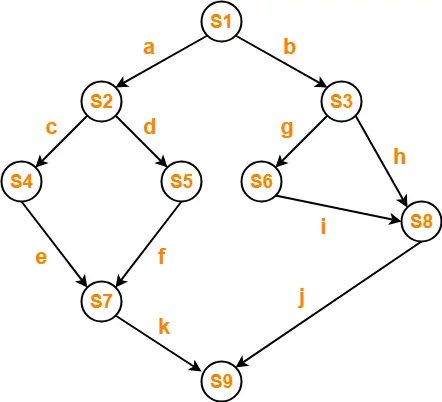## Problem-11:

Write a concurrent program using parbegin-parend and semaphores to represent the precedence constraints of the statements S1 to S6 as shown in given figure-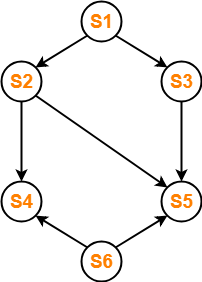## Solution-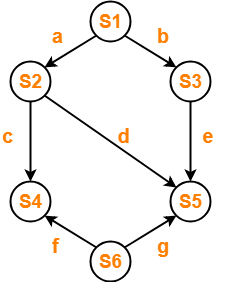```var
a,b,c,d,e,f,g : semaphore;
begin
cobegin
begin S1; V(a); V(b) end;
begin P(a); S2; V(c); V(d) end;
begin P(b); S3; V(e) end;
begin P(c); P(f); S4 end;
begin P(d); P(e); P(g); S5 end
begin S6; V(f); V(g) end;
coend
end;```

To watch video solutions and practice other problems,

Watch this Video Lecture

Get more notes and other study material of Operating System.

Watch video lectures by visiting our YouTube channel LearnVidFun.

## Semaphores in OS-

Before you go through this article, make sure that you have gone through the previous article on Semaphores in OS.

We have discussed-

• A semaphore is a simple integer variable used to provide synchronization among the processes.
• There are mainly two types of semaphores-## Binary Semaphores-

A binary semaphore is implemented as-

```struct semaphore
{
enum value (0,1);
Queue type L;
}
Wait (semaphore s)
{
if (s.value == 1)
{
s.value=0;
}
else
{
put process (PCB) in s.L;
sleep();
}
}
Signal (semaphore s)
{
if (s.L is empty)
{
s.value=1;
}
else
{
select a process (PCB) from s.L;
wake up();
}
}```

## Explanation-

The above implementation of binary semaphore has been explained in the following points-

## Point-01:

A binary semaphore has two components-

• An integer value which can be either 0 or 1
• An associated waiting list (usually a queue)

## Point-02:

• The waiting list of binary semaphore contains the processes that got blocked when trying to enter the critical section.
• In waiting list, the blocked processes are put to sleep.
• The waiting list is usually implemented using a queue data structure.
• Using a queue as waiting list ensures bounded waiting.
• This is because the process which arrives first in the waiting queue gets the chance to enter the critical section first.

## Point-03:

• The wait operation is executed when a process tries to enter the critical section.
• Then, there are two cases possible-

### Case-01: Binary Semaphore Value = 1

If the value of binary semaphore is 1,

• The value of binary semaphore is set to 0.
• The process is allowed to enter the critical section.

### Case-02: Binary Semaphore Value = 0

If the value of binary semaphore is 0,

• The process is blocked and not allowed to enter the critical section.
• The process is put to sleep in the waiting list.

## Point-04:

• The signal operation is executed when a process takes exit from the critical section.
• Then, there are two cases possible-

### Case-01: Waiting List is Empty-

• If the waiting list is empty, the value of binary semaphore is set to 1.

### Case-02: Waiting List is Not Empty-

• If the waiting list is not empty, a process is chosen from the waiting list and wake up to execute.

## Point-05:

Binary semaphores are mainly used for two purposes-

• To ensure mutual exclusion.
• To implement the order in which the processes must execute.

### Example-

 Wait (S1) Process P1 Signal (S2) Process P2 Signal (S1) Wait (S2) Process P3

In this example-

• Two binary semaphores S1 and S2 both initialized with 0 are used.
• The execution order of the processes is P2 → P1 → P3.

To gain better understanding about Binary Semaphores,

Watch this Video Lecture

Next Article- Practice Problems On Binary Semaphores

Get more notes and other study material of Operating System.

Watch video lectures by visiting our YouTube channel LearnVidFun.

## Semaphore in OS-

Before you go through this article, make sure that you have gone through the previous article on Semaphores in OS.

We have discussed-

• A semaphore is a simple integer variable used to provide synchronization among the processes.
• There are mainly two types of semaphores-In this article, we will discuss practice problems based on counting semaphores.

## Problem-01:

A counting semaphore S is initialized to 10. Then, 6 P operations and 4 V operations are performed on S. What is the final value of S?

## Solution-

We know-

• P operation also called as wait operation decrements the value of semaphore variable by 1.
• V operation also called as signal operation increments the value of semaphore variable by 1.

Thus,

Final value of semaphore variable S

= 10 – (6 x 1) + (4 x 1)

= 10 – 6 + 4

= 8

## Problem-02:

A counting semaphore S is initialized to 7. Then, 20 P operations and 15 V operations are performed on S. What is the final value of S?

## Solution-

We know-

• P operation also called as wait operation decrements the value of semaphore variable by 1.
• V operation also called as signal operation increments the value of semaphore variable by 1.

Thus,

Final value of semaphore variable S

= 7 – (20 x 1) + (15 x 1)

= 7 – 20 + 15

= 2

## Problem-03:

A shared variable x, initialized to zero, is operated on by four concurrent processes W, X, Y, Z as follows. Each of the processes W and X reads x from memory, increments by one, stores it to memory and then terminates. Each of the processes Y and Z reads x from memory, decrements by two, stores it to memory, and then terminates. Each process before reading x invokes the P operation (i.e. wait) on a counting semaphore S and invokes the V operation (i.e. signal) on the semaphore S after storing x to memory. Semaphore S is initialized to two. What is the maximum possible value of x after all processes complete execution?

1. -2
2. -1
3. 1
4. 2

## Solution-

The given question may be pictorially represented as-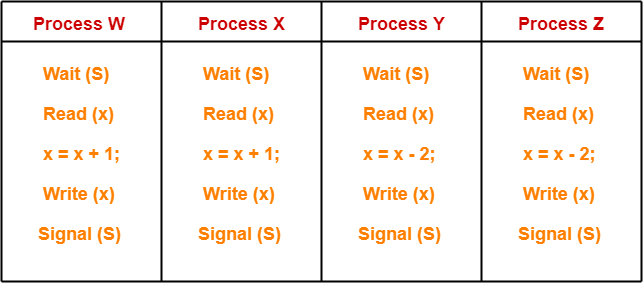Initially, counting semaphore S is initialized with value 2.

Now, We have been asked the maximum possible value of x after all the processes complete execution.

Clearly,

• Processes W and X increments the value of x.
• Processes Y and Z decrements the value of x.

To obtain the maximum value of x, the processes must execute in such a way that-

• Only the impact of the processes W and X remains on the value of x.
• The impact of processes Y and Z gets lost on the value of x.

### STRATEGY

• First of all, make the process W read the value of x = 0.
• Then, preempt the process W.
• Now, schedule process Y and process Z to execute one by one.
• After executing them, reschedule process W.
• Now, when process W gets scheduled again, it starts with value x = 0 and increments this value.
• This is because before preemption it had read the value x = 0.
• The updates from the processes Y and Z gets lost.
• Later, execute process X which again increments the value of x.
• Here, the lost update problem has been exploited.

This is achieved as-

### Step-01:

• Process W arrives.
• It executes the wait(S) operation and the value of S decrements by 1. Now, S = 1.
• It reads the value x = 0.
• It gets preempted.

### Step-02:

• Process Y arrives.
• It executes the wait(S) operation and the value of S decrements by 1. Now, S = 0.
• It reads the value x = 0.
• It decrements the value of x by 2. Now, x = 0 – 2 = -2.
• It writes the value x = -2 in the memory.
• It executes the signal(S) operation and the value of S increments by 1. Now, S = 1.
• Now, execution of process Y is completed.

### Step-03:

• Process Z arrives.
• It executes the wait(S) operation and the value of S decrements by 1. Now, S = 0.
• It reads the value x = -2.
• It decrements the value of x by 2. Now, x = – 2 – 2 = -4.
• It writes the value x = -4 in the memory.
• It executes the signal(S) operation and the value of S increments by 1. Now, S = 1.
• Now, execution of process Z is completed.

### Step-04:

• Process W gets scheduled again.
• It resumes its execution from where it left.
• Now, it increments the value of x by 1. Now, x = 0 + 1 = 1.
• It writes the value x = 1 in the memory.
• It executes the signal(S) operation and the value of S increments by 1. Now, S = 2.
• Now, execution of process W is completed.

### Step-05:

• Process X arrives.
• It executes the wait(S) operation and the value of S decrements by 1. Now, S = 1.
• It reads the value x = 1.
• It increments the value of x by 1. Now, x = 1 + 1 = 2.
• It writes the value x = 2 in the memory.
• It executes the signal(S) operation and the value of S increments by 1. Now, S = 2.
• Now, execution of process X is completed.

Thus,

• Final value of x = 2.
• This is the maximum possible value of x that can be achieved after executing all the 4 processes.
• Option (D) is correct.

## Problem-04:

In problem-03, what is the minimum possible value of x after all processes complete execution?

1. -4
2. -2
3. 2
4. 4

## Solution-

To obtain the minimum value of x, the processes must execute in such a way that-

• Only the impact of the processes Y and Z remains on the value of x.
• The impact of processes W and X gets lost on the value of x.

This can be achieved if processes execute in the following manner-

### Step-01:

• Process Y arrives.
• It executes the wait(S) operation and the value of S decrements by 1. Now, S = 1.
• It reads the value x = 0.
• It gets preempted.

### Step-02:

• Process W arrives.
• It executes the wait(S) operation and the value of S decrements by 1. Now, S = 0.
• It reads the value x = 0.
• It increments the value of x by 1. Now, x = 0 + 1 = 1.
• It writes the value x = 1 in the memory.
• It executes the signal(S) operation and the value of S increments by 1. Now, S = 1.
• Now, execution of process W is completed.

### Step-03:

• Process X arrives.
• It executes the wait(S) operation and the value of S decrements by 1. Now, S = 0.
• It reads the value x = 1.
• It increments the value of x by 1. Now, x = 1 + 1 = 2.
• It writes the value x = 2 in the memory.
• It executes the signal(S) operation and the value of S increments by 1. Now, S = 1.
• Now, execution of process X is completed.

### Step-04:

• Process Y gets scheduled again.
• It resumes its execution from where it left.
• Now, it decrements the value of x by 2. Now, x = 0 – 2 = -2.
• It writes the value x = -2 in the memory.
• It executes the signal(S) operation and the value of S increments by 1. Now, S = 2.
• Now, execution of process Y is completed.

### Step-05:

• Process Z arrives.
• It executes the wait(S) operation and the value of S decrements by 1. Now, S = 1.
• It reads the value x = -2.
• It decrements the value of x by 2. Now, x = -2 – 2 = -4.
• It writes the value x = -4 in the memory.
• It executes the signal(S) operation and the value of S increments by 1. Now, S = 2.
• Now, execution of process Z is completed.

Thus,

• Final value of x = -4.
• This is the minimum possible value of x that can be achieved after executing all the 4 processes.

Thus, Option (A) is correct.

To watch video solutions and practice other problems,

Watch this Video Lecture

Next Article- Binary Semaphores

Get more notes and other study material of Operating System.

Watch video lectures by visiting our YouTube channel LearnVidFun.

## Process Synchronization-

Before you go through this article, make sure that you have gone through the previous article on Process Synchronization.

We have discussed-

• Process Synchronization provides a synchronization among the processes.
• Synchronization mechanisms allow the processes to access critical section in a synchronized manner.
• This avoids the inconsistent results.

## Semaphores in OS-

• A semaphore is a simple integer variable.
• It is used to provide synchronization among multiple processes running concurrently.

## Types of Semaphores-

There are mainly two types of semaphores-1. Counting Semaphores
2. Binary Semaphores or Mutexes

## Counting Semaphores-

A counting semaphore is implemented as-

```struct semaphore
{
int value;
Queue type L;
}
Wait (semaphore s)
{
s.value = s.value - 1;
if (s.value < 0)
{
put process (PCB) in L;
sleep();
}
else
return;
}
Signal (semaphore s)
{
s.value = s.value + 1;
if (s.value <=0 )
{
select a process (PCB) from L;
wake up();
}
}```

## Explanation-

The above implementation of counting semaphore has been explained in the following points-

## Point-01:

A counting semaphore has two components-

• An integer value
• An associated waiting list (usually a queue)

## Point-02:

The value of counting semaphore may be positive or negative.

• Positive value indicates the number of processes that can be present in the critical section at the same time.
• Negative value indicates the number of processes that are blocked in the waiting list.

## Point-03:

• The waiting list of counting semaphore contains the processes that got blocked when trying to enter the critical section.
• In waiting list, the blocked processes are put to sleep.
• The waiting list is usually implemented using a queue data structure.
• Using a queue as waiting list ensures bounded waiting.
• This is because the process which arrives first in the waiting queue gets the chance to enter the critical section first.

## Point-04:

• The wait operation is executed when a process tries to enter the critical section.
• Wait operation decrements the value of counting semaphore by 1.

Then, following two cases are possible-

### Case-01: Counting Semaphore Value >= 0

• If the resulting value of counting semaphore is greater than or equal to 0, process is allowed to enter the critical section.

### Case-02: Counting Semaphore Value < 0

• If the resulting value of counting semaphore is less than 0, process is not allowed to enter the critical section.
• In this case, process is put to sleep in the waiting list.

## Point-05:

• The signal operation is executed when a process takes exit from the critical section.
• Signal operation increments the value of counting semaphore by 1.

Then, following two cases are possible-

### Case-01: Counting Semaphore <= 0

• If the resulting value of counting semaphore is less than or equal to 0, a process is chosen from the waiting list and wake up to execute.

### Case-02: Counting Semaphore > 0

• If the resulting value of counting semaphore is greater than 0, no action is taken.

## Point-06:

• By adjusting the value of counting semaphore, the number of processes that can enter the critical section can be adjusted.
• If the value of counting semaphore is initialized with N, then maximum N processes can be present in the critical section at any given time.

## Point-07:

• To implement mutual exclusion, the value of counting semaphore is initialized with 1.
• It ensures that only one process can be present in the critical section at any given time.

## Point-08:

In a system,

• Consider n units of a particular non-shareable resource are available.
• Then, n processes can use these n units at the same time.
• So, the access to these units is kept in the critical section.
• The value of counting semaphore is initialized with ‘n’.
• When a process enters the critical section, the value of counting semaphore decrements by 1.
• When a process exits the critical section, the value of counting semaphore increments by 1.

 In such scenarios, value of counting semaphore is initialized with value greater than 1.

## Point-09:

• Other names by which wait operation may be referred : Down operation, P operation.
• Other names by which signal operation may be referred : Up operation, V operation, Release operation.

To gain better understanding about Counting Semaphores,

Watch this Video Lecture

Next Article- Practice Problems On Counting Semaphores

Get more notes and other study material of Operating System.

Watch video lectures by visiting our YouTube channel LearnVidFun.

## Contiguous Memory Allocation-

Before you go through this article, make sure that you have gone through the previous articles on Static Partitioning and Dynamic Partitioning.

There are two popular techniques used for contiguous memory allocation-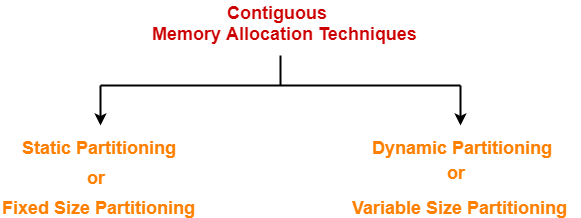In this article, we will discuss practice problems based on Contiguous Memory Allocation.

## Problem-01:

Consider six memory partitions of size 200 KB, 400 KB, 600 KB, 500 KB, 300 KB and 250 KB. These partitions need to be allocated to four processes of sizes 357 KB, 210 KB, 468 KB and 491 KB in that order.

Perform the allocation of processes using-

1. First Fit Algorithm
2. Best Fit Algorithm
3. Worst Fit Algorithm

## Solution-

According to question,

The main memory has been divided into fixed size partitions as-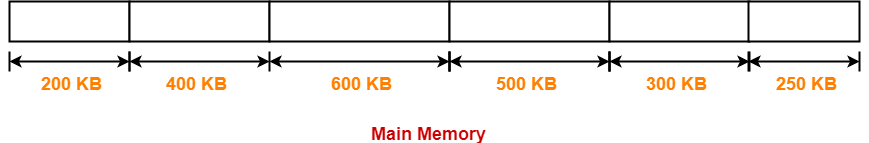Let us say the given processes are-

• Process P1 = 357 KB
• Process P2 = 210 KB
• Process P3 = 468 KB
• Process P4 = 491 KB

## Allocation Using First Fit Algorithm-

In First Fit Algorithm,

• Algorithm starts scanning the partitions serially.
• When a partition big enough to store the process is found, it allocates that partition to the process.

The allocation of partitions to the given processes is shown below-

### Step-01: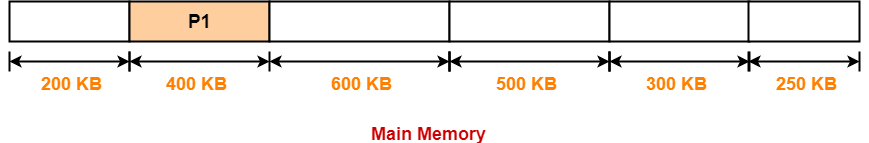### Step-02: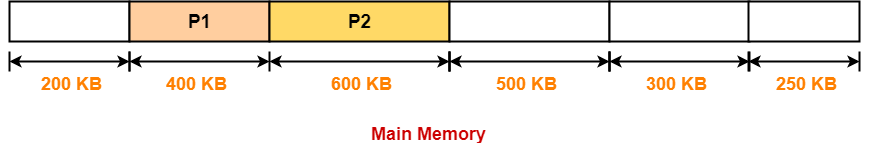### Step-03: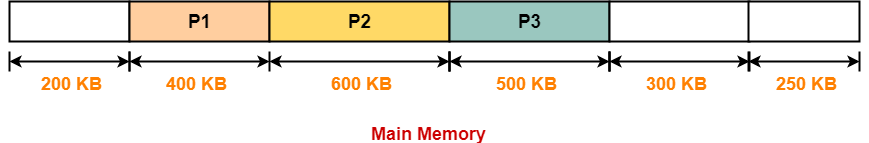### Step-04:

• Process P4 can not be allocated the memory.
• This is because no partition of size greater than or equal to the size of process P4 is available.

## Allocation Using Best Fit Algorithm-

In Best Fit Algorithm,

• Algorithm first scans all the partitions.
• It then allocates the partition of smallest size that can store the process.

The allocation of partitions to the given processes is shown below-

### Step-01:### Step-02: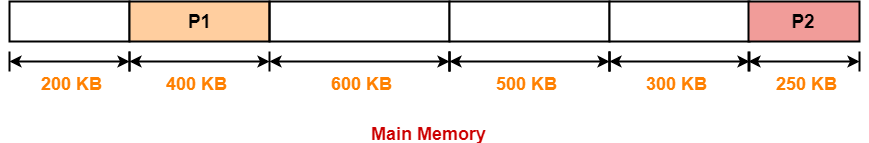### Step-03: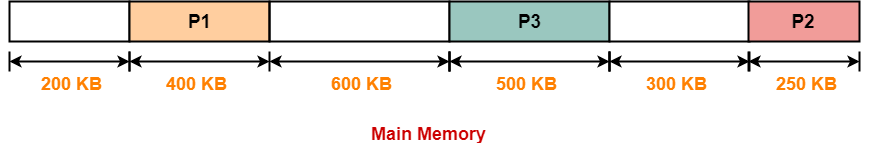### Step-04: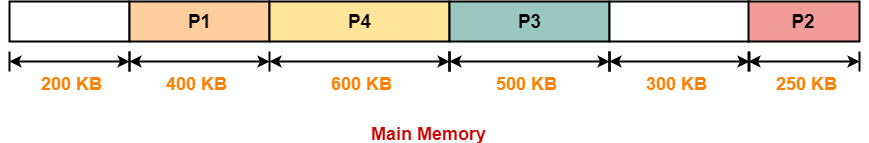## Allocation Using Worst Fit Algorithm-

In Worst Fit Algorithm,

• Algorithm first scans all the partitions.
• It then allocates the partition of largest size to the process.

The allocation of partitions to the given processes is shown below-

### Step-01: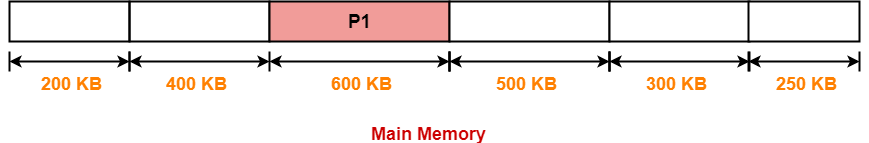### Step-02: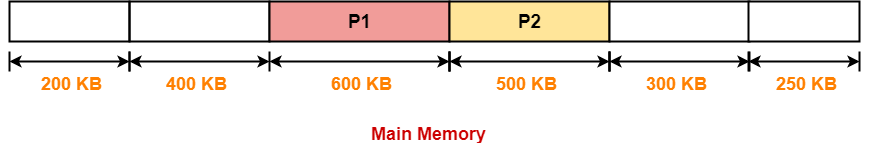### Step-03:

• Process P3 and Process P4 can not be allocated the memory.
• This is because no partition of size greater than or equal to the size of process P3 and process P4 is available.

## Problem-02:

Consider the following heap (figure) in which blank regions are not in use and hatched regions are in use-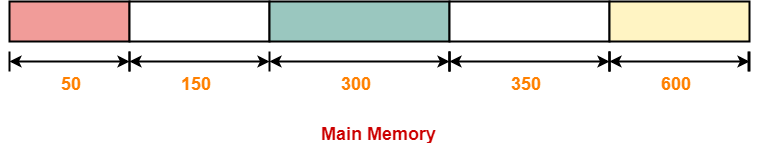The sequence of requests for blocks of size 300, 25, 125, 50 can be satisfied if we use-

1. Either first fit or best fit policy (any one)
2. First fit but not best fit policy
3. Best fit but not first fit policy
4. None of the above

## Solution-

The allocation follows variable size partitioning scheme.

Let us say the given processes are-

• Process P1 = 300 units
• Process P2 = 25 units
• Process P3 = 125 units
• Process P4 = 50 units

## Allocation Using First Fit Algorithm-

The allocation of partitions to the given processes is shown below-

### Step-01: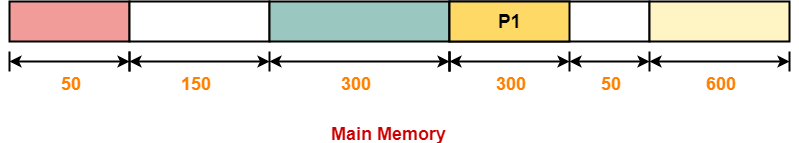### Step-02: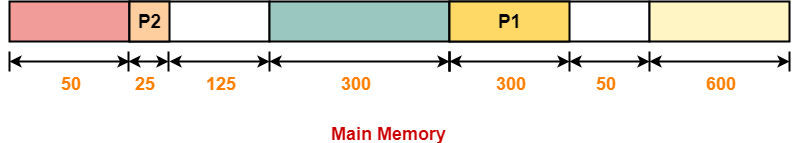### Step-03: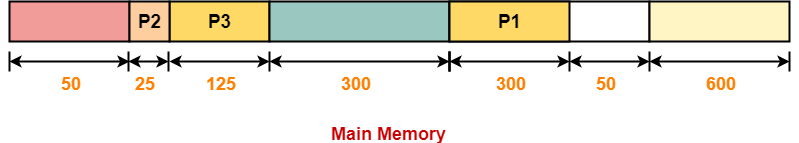### Step-04: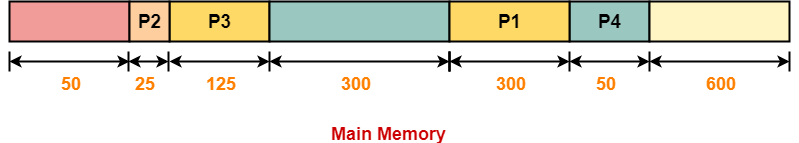## Allocation Using Best Fit Algorithm-

The allocation of partitions to the given processes is shown below-

### Step-01:### Step-02: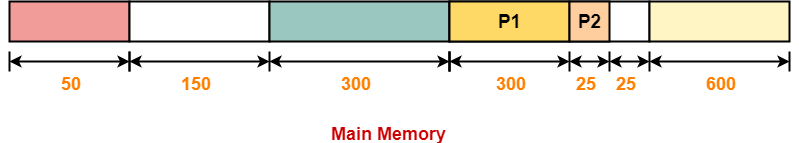### Step-03: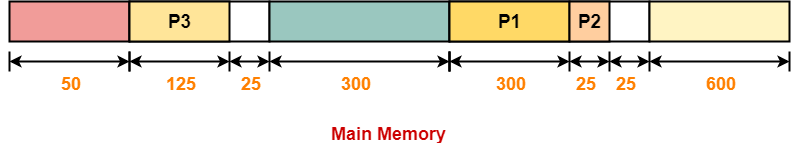### Step-04:

• Process P4 can not be allocated the memory.
• This is because no partition of size greater than or equal to the size of process P4 is available.

Thus,

• Only first fit allocation policy succeeds in allocating memory to all the processes.
• Option (B) is correct.